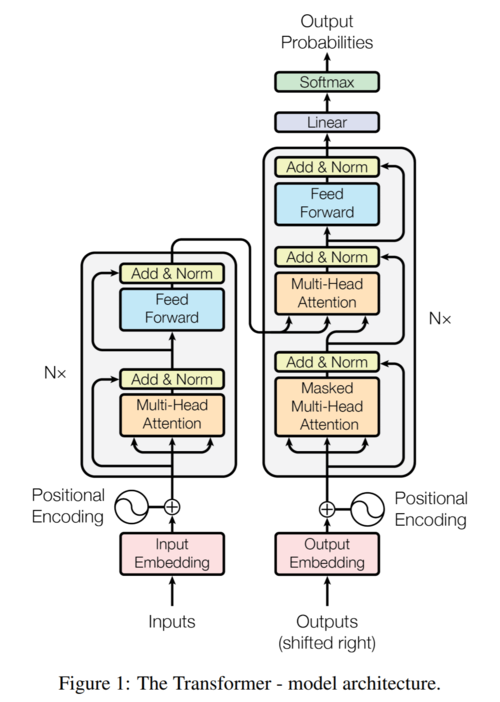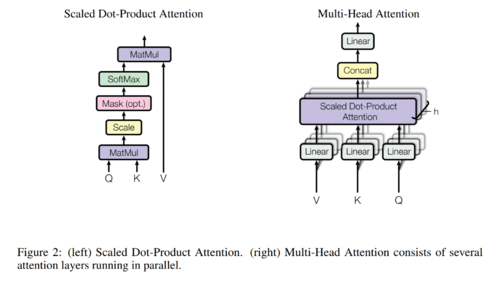# Transformer (machine learning model)

$$\newcommand{\P}[]{\unicode{xB6}} \newcommand{\AA}[]{\unicode{x212B}} \newcommand{\empty}[]{\emptyset} \newcommand{\O}[]{\emptyset} \newcommand{\Alpha}[]{Α} \newcommand{\Beta}[]{Β} \newcommand{\Epsilon}[]{Ε} \newcommand{\Iota}[]{Ι} \newcommand{\Kappa}[]{Κ} \newcommand{\Rho}[]{Ρ} \newcommand{\Tau}[]{Τ} \newcommand{\Zeta}[]{Ζ} \newcommand{\Mu}[]{\unicode{x039C}} \newcommand{\Chi}[]{Χ} \newcommand{\Eta}[]{\unicode{x0397}} \newcommand{\Nu}[]{\unicode{x039D}} \newcommand{\Omicron}[]{\unicode{x039F}} \DeclareMathOperator{\sgn}{sgn} \def\oiint{\mathop{\vcenter{\mathchoice{\huge\unicode{x222F}\,}{\unicode{x222F}}{\unicode{x222F}}{\unicode{x222F}}}\,}\nolimits} \def\oiiint{\mathop{\vcenter{\mathchoice{\huge\unicode{x2230}\,}{\unicode{x2230}}{\unicode{x2230}}{\unicode{x2230}}}\,}\nolimits}$$

Attention is all you need paper
A neural network architecture by Google.
It is currently the best at NLP tasks and has mostly replaced RNNs for these tasks.

## Architecture

The Transformer uses an encoder-decoder architecture. Both the encoder and decoder are comprised of multiple identical layers which have attention and feedforward sublayers.### Positional Encoding

The positional encoding is a sine wave which is added (not concatenated) to the word embeddings. See the original paper for details.
Today, people use learned embeddings.

### Attention

Attention is the main contribution of the transformer architecture.The attention block outputs a weighted average of values in a dictionary of key-value pairs.
In the image above:

• $$\displaystyle Q$$ represents queries (each query is a vector)
• $$\displaystyle K$$ represents keys
• $$\displaystyle V$$ represents values

The attention block can be represented as the following equation:

• $$\displaystyle Z = \operatorname{SoftMax}(\frac{QK^T}{\sqrt{d_k}})V$$

Each word embedded gets its own key, query, and value vectors which are stacked alongside those of other words to form K, Q, and V matrices.
These K, Q, and V matrices are computed by multiplying the embedding matrix $$\displaystyle X$$ with weights $$\displaystyle W_{K}, W_{Q}, W_{V}$$.

In multi-headed attention, you have multiple $$\displaystyle W_{K}, W_{Q}, W_{V}$$ and get an output for each attention head $$\displaystyle Z_i$$.
These are concatenated and multiplied by another weight matrix to form the output $$\displaystyle Z$$, the input to the next layer of the block.

Self attention

The encoder and parts of the decoder, use self-attention which means the keys, values, and queries are all generated from the embedding.

Encoder-decoder attention

The decoder also uses encoder-decoder attention where the keys and values are from the output embedding of the encoder (i.e. the input sentence) but the queries are generated from the decoder input (i.e. the previously-generated output).

### Encoder

The entire encoder receives as input the input embedding added to a positional encoding.
The encoder is comprised of N=6 blocks, each with 2 layers.
Each block contains a multi-headed attention layer followed by a feed-forward layer.
The feed-forward layer is applied to each word individually.
Each of the two layers of the encoder is a residual layer with an add-and-normalize residual connection.

### Decoder

Each decoder consists of a self-attention, an encoder-decoder attention, and a feed-forward layer.
As with the encoder, each layer is followed by an add-and-normalize residual connection.
The encoder-decoder attention gets its keys and values from the output of the last encoder block.
The same keys and values are passed to all encoder-decoder layers rather than having each layer generate its own.

## Code

See Hugging Face which contains many pretrained transfromers such as Bert.

## Resources

Guides and explanations